Anzeige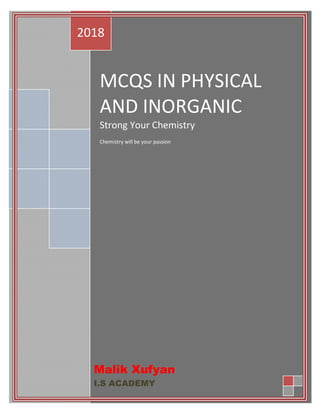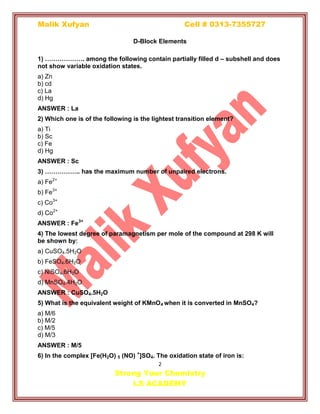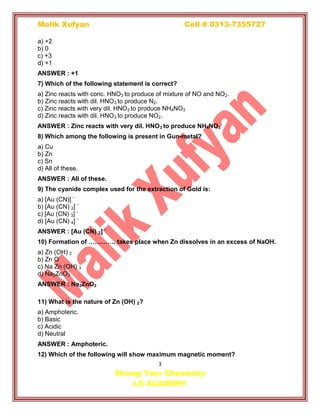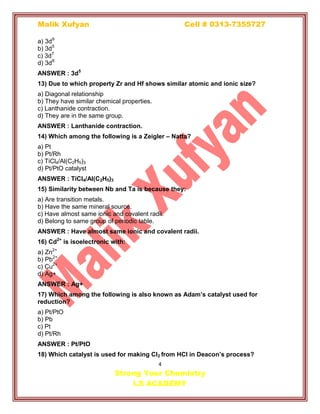Anzeige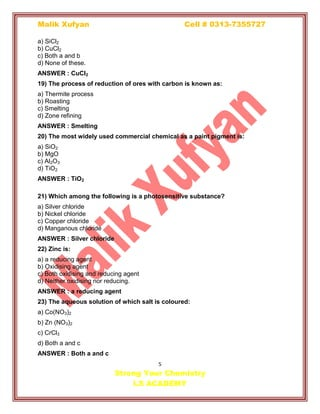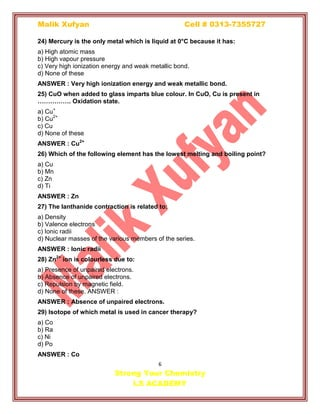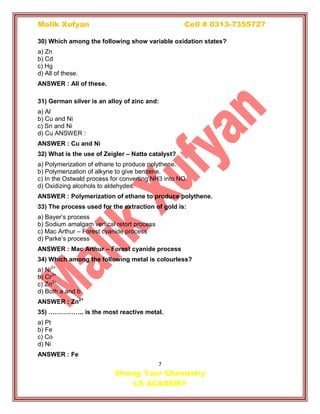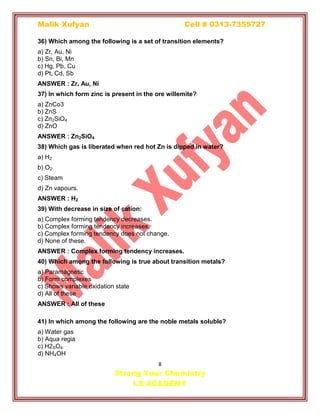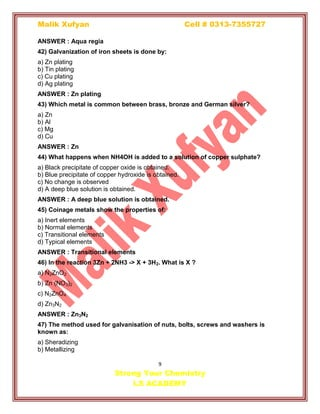Anzeige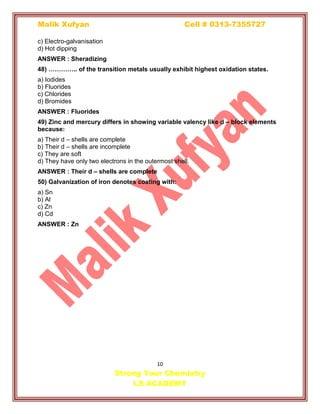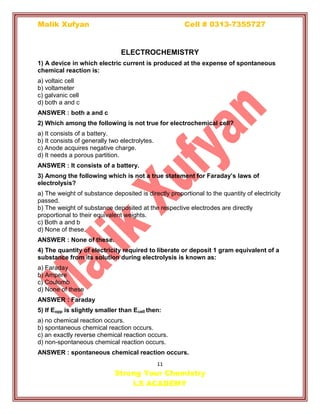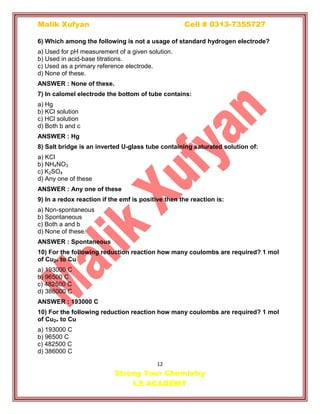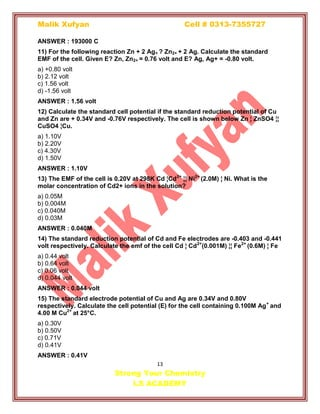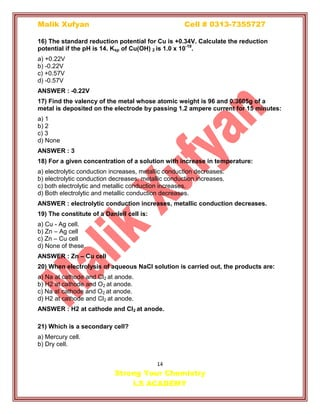Anzeige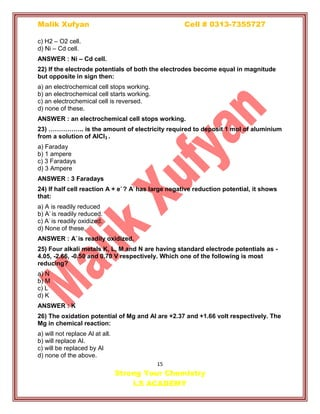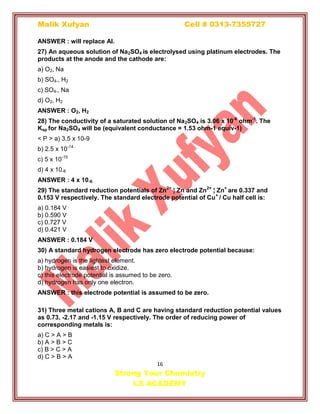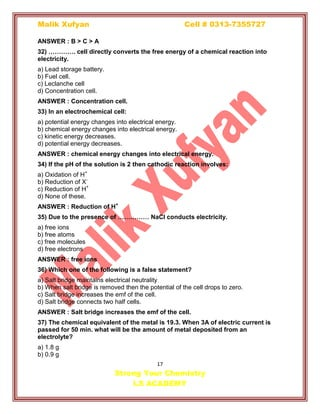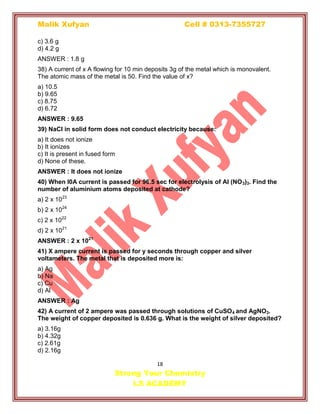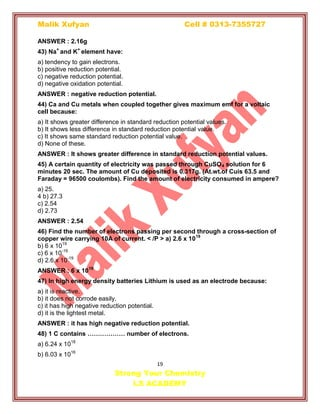Anzeige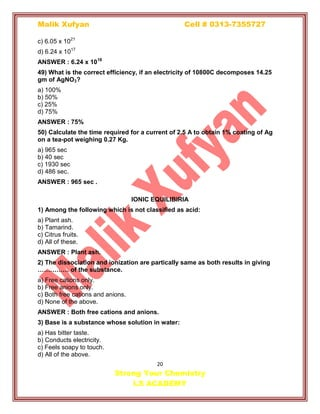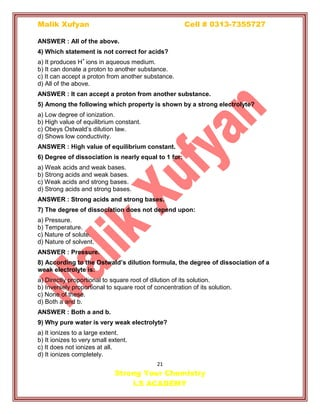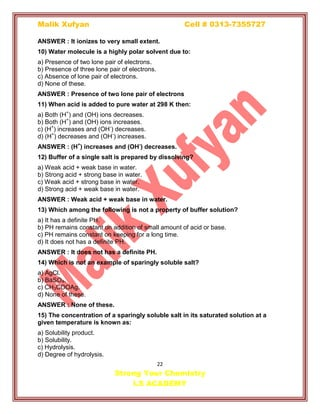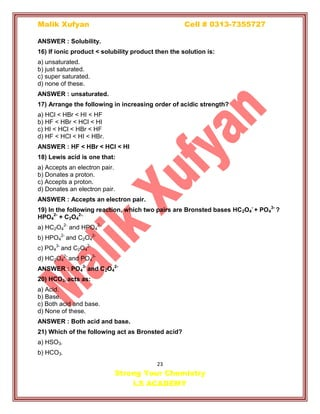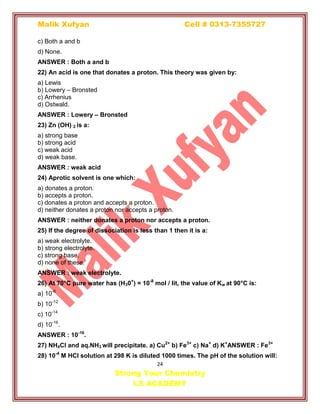Anzeige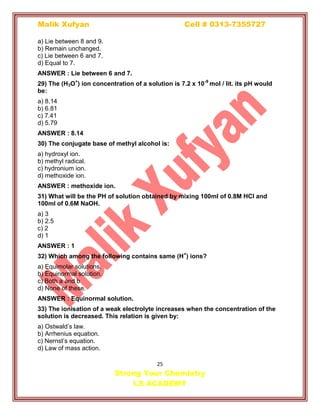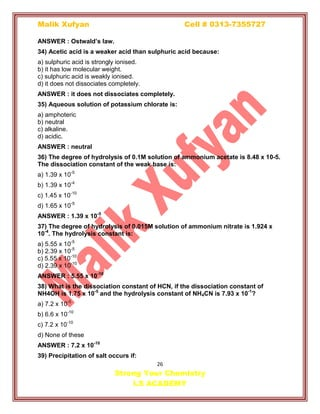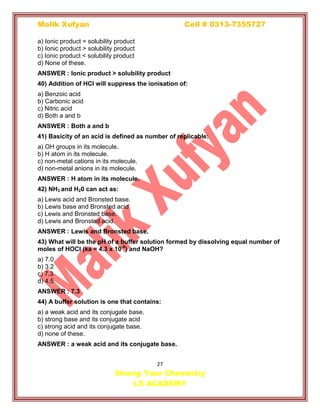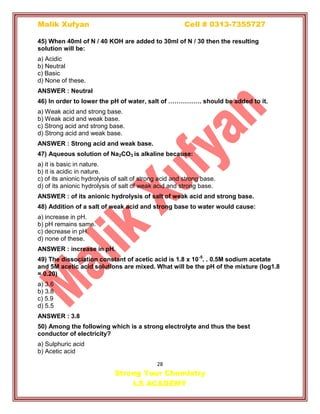Nächste SlideShare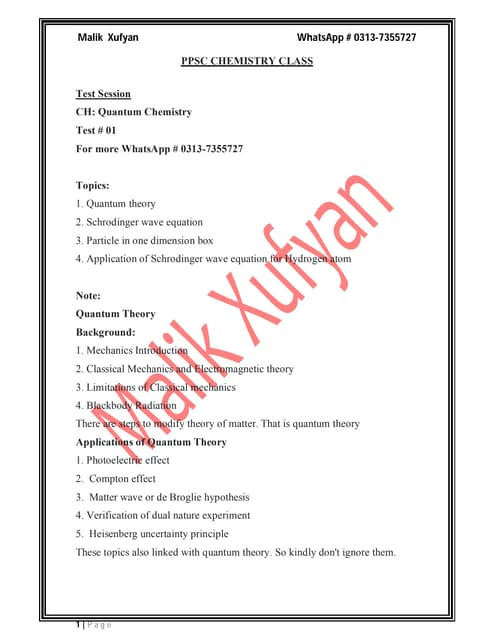PPSC Chemistry Lecturer Preparation (Test # 01)- Malik Xufyan
1 von 29
Anzeige

### Mcqs in inorganic and physical chemistry By Malik Xufyan

1. MCQS IN PHYSICAL AND INORGANIC Strong Your Chemistry Chemistry will be your passion 2018 Malik Xufyan I.S ACADEMY
2. Malik Xufyan Cell # 0313-7355727 2 Strong Your Chemistry I.S ACADEMY D-Block Elements 1) ………………. among the following contain partially filled d – subshell and does not show variable oxidation states. a) Zn b) cd c) La d) Hg ANSWER : La 2) Which one is of the following is the lightest transition element? a) Ti b) Sc c) Fe d) Hg ANSWER : Sc 3) …………….. has the maximum number of unpaired electrons. a) Fe2+ b) Fe3+ c) Co3+ d) Co2+ ANSWER : Fe3+ 4) The lowest degree of paramagnetism per mole of the compound at 298 K will be shown by: a) CuSO4.5H2O b) FeSO4.6H2O c) NiSO4.6H2O d) MnSO4.4H2O ANSWER : CuSO4.5H2O 5) What is the equivalent weight of KMnO4 when it is converted in MnSO4? a) M/6 b) M/2 c) M/5 d) M/3 ANSWER : M/5 6) In the complex [Fe(H2O) 5 (NO) + ]SO4. The oxidation state of iron is:
3. Malik Xufyan Cell # 0313-7355727 3 Strong Your Chemistry I.S ACADEMY a) +2 b) 0 c) +3 d) +1 ANSWER : +1 7) Which of the following statement is correct? a) Zinc reacts with conc. HNO3 to produce of mixture of NO and NO2. b) Zinc reacts with dil. HNO3 to produce N2. c) Zinc reacts with very dil. HNO3 to produce NH4NO3 d) Zinc reacts with dil. HNO3 to produce NO2. ANSWER : Zinc reacts with very dil. HNO3 to produce NH4NO3 8) Which among the following is present in Gun-metal? a) Cu b) Zn c) Sn d) All of these. ANSWER : All of these. 9) The cyanide complex used for the extraction of Gold is: a) [Au (CN)] - b) [Au (CN) 2] - c) [Au (CN) 3] - d) [Au (CN) 4] - ANSWER : [Au (CN) 2] - 10) Formation of …………. takes place when Zn dissolves in an excess of NaOH. a) Zn (OH) 2 b) Zn O c) Na Zn (OH) 3 d) Na2ZnO2 ANSWER : Na2ZnO2 11) What is the nature of Zn (OH) 2? a) Amphoteric. b) Basic c) Acidic d) Neutral ANSWER : Amphoteric. 12) Which of the following will show maximum magnetic moment?
5. Malik Xufyan Cell # 0313-7355727 5 Strong Your Chemistry I.S ACADEMY a) SiCl2 b) CuCl2 c) Both a and b d) None of these. ANSWER : CuCl2 19) The process of reduction of ores with carbon is known as: a) Thermite process b) Roasting c) Smelting d) Zone refining ANSWER : Smelting 20) The most widely used commercial chemical as a paint pigment is: a) SiO2 b) MgO c) Al2O3 d) TiO2 ANSWER : TiO2 21) Which among the following is a photosensitive substance? a) Silver chloride b) Nickel chloride c) Copper chloride d) Manganous chloride ANSWER : Silver chloride 22) Zinc is: a) a reducing agent b) Oxidising agent c) Both oxidising and reducing agent d) Neither oxidising nor reducing. ANSWER : a reducing agent 23) The aqueous solution of which salt is coloured: a) Co(NO3)2 b) Zn (NO3)2 c) CrCl3 d) Both a and c ANSWER : Both a and c
7. Malik Xufyan Cell # 0313-7355727 7 Strong Your Chemistry I.S ACADEMY 30) Which among the following show variable oxidation states? a) Zn b) Cd c) Hg d) All of these. ANSWER : All of these. 31) German silver is an alloy of zinc and: a) Al b) Cu and Ni c) Sn and Ni d) Cu ANSWER : ANSWER : Cu and Ni 32) What is the use of Zeigler – Natta catalyst? a) Polymerization of ethane to produce polythene. b) Polymerization of alkyne to give benzene. c) In the Ostwald process for converting NH3 into NO. d) Oxidizing alcohols to aldehydes. ANSWER : Polymerization of ethane to produce polythene. 33) The process used for the extraction of gold is: a) Bayer’s process b) Sodium amalgam vertical retort process c) Mac Arthur – Forest cyanide process d) Parke’s process ANSWER : Mac Arthur – Forest cyanide process 34) Which among the following metal is colourless? a) Ni2+ b) Cr3+ c) Zn2+ d) Both a and b ANSWER : Zn2+ 35) …………….. is the most reactive metal. a) Pt b) Fe c) Co d) Ni ANSWER : Fe
8. Malik Xufyan Cell # 0313-7355727 8 Strong Your Chemistry I.S ACADEMY 36) Which among the following is a set of transition elements? a) Zr, Au, Ni b) Sn, Bi, Mn c) Hg, Pb, Cu d) Pt, Cd, Sb ANSWER : Zr, Au, Ni 37) In which form zinc is present in the ore willemite? a) ZnCo3 b) ZnS c) Zn2SiO4 d) ZnO ANSWER : Zn2SiO4 38) Which gas is liberated when red hot Zn is dipped in water? a) H2 b) O2 c) Steam d) Zn vapours. ANSWER : H2 39) With decrease in size of cation: a) Complex forming tendency decreases. b) Complex forming tendency increases. c) Complex forming tendency does not change. d) None of these. ANSWER : Complex forming tendency increases. 40) Which among the following is true about transition metals? a) Paramagnetic b) Form complexes c) Shows variable oxidation state d) All of these ANSWER : All of these 41) In which among the following are the noble metals soluble? a) Water gas b) Aqua regia c) H2SO4 d) NH4OH
9. Malik Xufyan Cell # 0313-7355727 9 Strong Your Chemistry I.S ACADEMY ANSWER : Aqua regia 42) Galvanization of iron sheets is done by: a) Zn plating b) Tin plating c) Cu plating d) Ag plating ANSWER : Zn plating 43) Which metal is common between brass, bronze and German silver? a) Zn b) Al c) Mg d) Cu ANSWER : Zn 44) What happens when NH4OH is added to a solution of copper sulphate? a) Black precipitate of copper oxide is obtained. b) Blue precipitate of copper hydroxide is obtained. c) No change is observed d) A deep blue solution is obtained. ANSWER : A deep blue solution is obtained. 45) Coinage metals show the properties of: a) Inert elements b) Normal elements c) Transitional elements d) Typical elements ANSWER : Transitional elements 46) In the reaction 3Zn + 2NH3 -> X + 3H2. What is X ? a) N2ZnO2 b) Zn (NO3)2 c) N2ZnO4 d) Zn3N2 ANSWER : Zn3N2 47) The method used for galvanisation of nuts, bolts, screws and washers is known as: a) Sheradizing b) Metallizing
10. Malik Xufyan Cell # 0313-7355727 10 Strong Your Chemistry I.S ACADEMY c) Electro-galvanisation d) Hot dipping ANSWER : Sheradizing 48) ………….. of the transition metals usually exhibit highest oxidation states. a) Iodides b) Fluorides c) Chlorides d) Bromides ANSWER : Fluorides 49) Zinc and mercury differs in showing variable valency like d – block elements because: a) Their d – shells are complete b) Their d – shells are incomplete c) They are soft d) They have only two electrons in the outermost shell. ANSWER : Their d – shells are complete 50) Galvanization of iron denotes coating with: a) Sn b) Al c) Zn d) Cd ANSWER : Zn
11. Malik Xufyan Cell # 0313-7355727 11 Strong Your Chemistry I.S ACADEMY ELECTROCHEMISTRY 1) A device in which electric current is produced at the expense of spontaneous chemical reaction is: a) voltaic cell b) voltameter c) galvanic cell d) both a and c ANSWER : both a and c 2) Which among the following is not true for electrochemical cell? a) It consists of a battery. b) It consists of generally two electrolytes. c) Anode acquires negative charge. d) It needs a porous partition. ANSWER : It consists of a battery. 3) Among the following which is not a true statement for Faraday’s laws of electrolysis? a) The weight of substance deposited is directly proportional to the quantity of electricity passed. b) The weight of substance deposited at the respective electrodes are directly proportional to their equivalent weights. c) Both a and b d) None of these. ANSWER : None of these. 4) The quantity of electricity required to liberate or deposit 1 gram equivalent of a substance from its solution during electrolysis is known as: a) Faraday b) Ampere c) Coulomb d) None of these ANSWER : Faraday 5) If Eopp is slightly smaller than Ecell then: a) no chemical reaction occurs. b) spontaneous chemical reaction occurs. c) an exactly reverse chemical reaction occurs. d) non-spontaneous chemical reaction occurs. ANSWER : spontaneous chemical reaction occurs.
12. Malik Xufyan Cell # 0313-7355727 12 Strong Your Chemistry I.S ACADEMY 6) Which among the following is not a usage of standard hydrogen electrode? a) Used for pH measurement of a given solution. b) Used in acid-base titrations. c) Used as a primary reference electrode. d) None of these. ANSWER : None of these. 7) In calomel electrode the bottom of tube contains: a) Hg b) KCl solution c) HCl solution d) Both b and c ANSWER : Hg 8) Salt bridge is an inverted U-glass tube containing saturated solution of: a) KCl b) NH4NO3 c) K2SO4 d) Any one of these ANSWER : Any one of these 9) In a redox reaction if the emf is positive then the reaction is: a) Non-spontaneous b) Spontaneous c) Both a and b d) None of these. ANSWER : Spontaneous 10) For the following reduction reaction how many coulombs are required? 1 mol of Cu2+ to Cu a) 193000 C b) 96500 C c) 482500 C d) 386000 C ANSWER : 193000 C 10) For the following reduction reaction how many coulombs are required? 1 mol of Cu2+ to Cu a) 193000 C b) 96500 C c) 482500 C d) 386000 C
13. Malik Xufyan Cell # 0313-7355727 13 Strong Your Chemistry I.S ACADEMY ANSWER : 193000 C 11) For the following reaction Zn + 2 Ag+ ? Zn2+ + 2 Ag. Calculate the standard EMF of the cell. Given E? Zn, Zn2+ = 0.76 volt and E? Ag, Ag+ = -0.80 volt. a) +0.80 volt b) 2.12 volt c) 1.56 volt d) -1.56 volt ANSWER : 1.56 volt 12) Calculate the standard cell potential if the standard reduction potential of Cu and Zn are + 0.34V and -0.76V respectively. The cell is shown below Zn ¦ ZnSO4 ¦¦ CuSO4 ¦Cu. a) 1.10V b) 2.20V c) 4.30V d) 1.50V ANSWER : 1.10V 13) The EMF of the cell is 0.20V at 298K Cd ¦Cd2+ ¦¦ Ni2+ (2.0M) ¦ Ni. What is the molar concentration of Cd2+ ions in the solution? a) 0.05M b) 0.004M c) 0.040M d) 0.03M ANSWER : 0.040M 14) The standard reduction potential of Cd and Fe electrodes are -0.403 and -0.441 volt respectively. Calculate the emf of the cell Cd ¦ Cd2+ (0.001M) ¦¦ Fe2+ (0.6M) ¦ Fe a) 0.44 volt b) 0.64 volt c) 0.06 volt d) 0.044 volt ANSWER : 0.044 volt 15) The standard electrode potential of Cu and Ag are 0.34V and 0.80V respectively. Calculate the cell potential (E) for the cell containing 0.100M Ag+ and 4.00 M Cu2+ at 25°C. a) 0.30V b) 0.50V c) 0.71V d) 0.41V ANSWER : 0.41V
14. Malik Xufyan Cell # 0313-7355727 14 Strong Your Chemistry I.S ACADEMY 16) The standard reduction potential for Cu is +0.34V. Calculate the reduction potential if the pH is 14. Ksp of Cu(OH) 2 is 1.0 x 10-19 . a) +0.22V b) -0.22V c) +0.57V d) -0.57V ANSWER : -0.22V 17) Find the valency of the metal whose atomic weight is 96 and 0.3605g of a metal is deposited on the electrode by passing 1.2 ampere current for 15 minutes: a) 1 b) 2 c) 3 d) None ANSWER : 3 18) For a given concentration of a solution with increase in temperature: a) electrolytic conduction increases, metallic conduction decreases. b) electrolytic conduction decreases, metallic conduction increases. c) both electrolytic and metallic conduction increases. d) Both electrolytic and metallic conduction decreases. ANSWER : electrolytic conduction increases, metallic conduction decreases. 19) The constitute of a Danlell cell is: a) Cu - Ag cell. b) Zn – Ag cell c) Zn – Cu cell d) None of these. ANSWER : Zn – Cu cell 20) When electrolysis of aqueous NaCl solution is carried out, the products are: a) Na at cathode and Cl2 at anode. b) H2 at cathode and O2 at anode. c) Na at cathode and O2 at anode. d) H2 at cathode and Cl2 at anode. ANSWER : H2 at cathode and Cl2 at anode. 21) Which is a secondary cell? a) Mercury cell. b) Dry cell.
16. Malik Xufyan Cell # 0313-7355727 16 Strong Your Chemistry I.S ACADEMY ANSWER : will replace Al. 27) An aqueous solution of Na2SO4 is electrolysed using platinum electrodes. The products at the anode and the cathode are: a) O2, Na b) SO4-, H2 c) SO4-, Na d) O2, H2 ANSWER : O2, H2 28) The conductivity of a saturated solution of Na2SO4 is 3.06 x 10-6 ohm-1 . The Ksp for Na2SO4 will be (equivalent conductance = 1.53 ohm-1 equiv-1) < P > a) 3.5 x 10-9 b) 2.5 x 10-14 c) 5 x 10-15 d) 4 x 10-6 ANSWER : 4 x 10-6 29) The standard reduction potentials of Zn2+ ¦ Zn and Zn2+ ¦ Zn+ are 0.337 and 0.153 V respectively. The standard electrode potential of Cu+ / Cu half cell is: a) 0.184 V b) 0.590 V c) 0.727 V d) 0.421 V ANSWER : 0.184 V 30) A standard hydrogen electrode has zero electrode potential because: a) hydrogen is the lightest element. b) hydrogen is easiest to oxidize. c) this electrode potential is assumed to be zero. d) hydrogen has only one electron. ANSWER : this electrode potential is assumed to be zero. 31) Three metal cations A, B and C are having standard reduction potential values as 0.73, -2.17 and -1.15 V respectively. The order of reducing power of corresponding metals is: a) C > A > B b) A > B > C c) B > C > A d) C > B > A
17. Malik Xufyan Cell # 0313-7355727 17 Strong Your Chemistry I.S ACADEMY ANSWER : B > C > A 32) …………. cell directly converts the free energy of a chemical reaction into electricity. a) Lead storage battery. b) Fuel cell. c) Leclanche cell d) Concentration cell. ANSWER : Concentration cell. 33) In an electrochemical cell: a) potential energy changes into electrical energy. b) chemical energy changes into electrical energy. c) kinetic energy decreases. d) potential energy decreases. ANSWER : chemical energy changes into electrical energy. 34) If the pH of the solution is 2 then cathodic reaction involves: a) Oxidation of H+ b) Reduction of X- c) Reduction of H+ d) None of these. ANSWER : Reduction of H+ 35) Due to the presence of …………… NaCl conducts electricity. a) free ions b) free atoms c) free molecules d) free electrons ANSWER : free ions 36) Which one of the following is a false statement? a) Salt bridge maintains electrical neutrality b) When salt bridge is removed then the potential of the cell drops to zero. c) Salt bridge increases the emf of the cell. d) Salt bridge connects two half cells. ANSWER : Salt bridge increases the emf of the cell. 37) The chemical equivalent of the metal is 19.3. When 3A of electric current is passed for 50 min. what will be the amount of metal deposited from an electrolyte? a) 1.8 g b) 0.9 g
18. Malik Xufyan Cell # 0313-7355727 18 Strong Your Chemistry I.S ACADEMY c) 3.6 g d) 4.2 g ANSWER : 1.8 g 38) A current of x A flowing for 10 min deposits 3g of the metal which is monovalent. The atomic mass of the metal is 50. Find the value of x? a) 10.5 b) 9.65 c) 8.75 d) 6.72 ANSWER : 9.65 39) NaCl in solid form does not conduct electricity because: a) It does not ionize b) It ionizes c) It is present in fused form d) None of these. ANSWER : It does not ionize 40) When I0A current is passed for 96.5 sec for electrolysis of Al (NO3)3. Find the number of aluminium atoms deposited at cathode? a) 2 x 1023 b) 2 x 1024 c) 2 x 1022 d) 2 x 1021 ANSWER : 2 x 1021 41) X ampere current is passed for y seconds through copper and silver voltameters. The metal that is deposited more is: a) Ag b) Na c) Cu d) Al ANSWER : Ag 42) A current of 2 ampere was passed through solutions of CuSO4 and AgNO3. The weight of copper deposited is 0.636 g. What is the weight of silver deposited? a) 3.16g b) 4.32g c) 2.61g d) 2.16g
19. Malik Xufyan Cell # 0313-7355727 19 Strong Your Chemistry I.S ACADEMY ANSWER : 2.16g 43) Na+ and K+ element have: a) tendency to gain electrons. b) positive reduction potential. c) negative reduction potential. d) negative oxidation potential. ANSWER : negative reduction potential. 44) Ca and Cu metals when coupled together gives maximum emf for a voltaic cell because: a) It shows greater difference in standard reduction potential values. b) It shows less difference in standard reduction potential value. c) It shows same standard reduction potential value. d) None of these. ANSWER : It shows greater difference in standard reduction potential values. 45) A certain quantity of electricity was passed through CuSO4 solution for 6 minutes 20 sec. The amount of Cu deposited is 0.317g. (At.wt.of Cuis 63.5 and Faraday = 96500 coulombs). Find the amount of electricity consumed in ampere? a) 25. 4 b) 27.3 c) 2.54 d) 2.73 ANSWER : 2.54 46) Find the number of electrons passing per second through a cross-section of copper wire carrying 10A of current. < /P > a) 2.6 x 1019 b) 6 x 1019 c) 6 x 10-19 d) 2.6 x 10-19 ANSWER : 6 x 1019 47) In high energy density batteries Lithium is used as an electrode because: a) it is reactive. b) it does not corrode easily. c) it has high negative reduction potential. d) it is the lightest metal. ANSWER : it has high negative reduction potential. 48) 1 C contains ……………… number of electrons. a) 6.24 x 1018 b) 6.03 x 1016
20. Malik Xufyan Cell # 0313-7355727 20 Strong Your Chemistry I.S ACADEMY c) 6.05 x 1021 d) 6.24 x 1017 ANSWER : 6.24 x 1018 49) What is the correct efficiency, if an electricity of 10800C decomposes 14.25 gm of AgNO3? a) 100% b) 50% c) 25% d) 75% ANSWER : 75% 50) Calculate the time required for a current of 2.5 A to obtain 1% coating of Ag on a tea-pot weighing 0.27 Kg. a) 965 sec b) 40 sec c) 1930 sec d) 486 sec. ANSWER : 965 sec . IONIC EQUILIBIRIA 1) Among the following which is not classified as acid: a) Plant ash. b) Tamarind. c) Citrus fruits. d) All of these. ANSWER : Plant ash. 2) The dissociation and ionization are partically same as both results in giving …………… of the substance. a) Free cations only. b) Free anions only. c) Both free cations and anions. d) None of the above. ANSWER : Both free cations and anions. 3) Base is a substance whose solution in water: a) Has bitter taste. b) Conducts electricity. c) Feels soapy to touch. d) All of the above.
21. Malik Xufyan Cell # 0313-7355727 21 Strong Your Chemistry I.S ACADEMY ANSWER : All of the above. 4) Which statement is not correct for acids? a) It produces H+ ions in aqueous medium. b) It can donate a proton to another substance. c) It can accept a proton from another substance. d) All of the above. ANSWER : It can accept a proton from another substance. 5) Among the following which property is shown by a strong electrolyte? a) Low degree of ionization. b) High value of equilibrium constant. c) Obeys Ostwald’s dilution law. d) Shows low conductivity. ANSWER : High value of equilibrium constant. 6) Degree of dissociation is nearly equal to 1 for: a) Weak acids and weak bases. b) Strong acids and weak bases. c) Weak acids and strong bases. d) Strong acids and strong bases. ANSWER : Strong acids and strong bases. 7) The degree of dissociation does not depend upon: a) Pressure. b) Temperature. c) Nature of solute. d) Nature of solvent. ANSWER : Pressure. 8) According to the Ostwald’s dilution formula, the degree of dissociation of a weak electrolyte is: a) Directly proportional to square root of dilution of its solution. b) Inversely proportional to square root of concentration of its solution. c) None of these. d) Both a and b. ANSWER : Both a and b. 9) Why pure water is very weak electrolyte? a) It ionizes to a large extent. b) It ionizes to very small extent. c) It does not ionizes at all. d) It ionizes completely.
22. Malik Xufyan Cell # 0313-7355727 22 Strong Your Chemistry I.S ACADEMY ANSWER : It ionizes to very small extent. 10) Water molecule is a highly polar solvent due to: a) Presence of two lone pair of electrons. b) Presence of three lone pair of electrons. c) Absence of lone pair of electrons. d) None of these. ANSWER : Presence of two lone pair of electrons 11) When acid is added to pure water at 298 K then: a) Both (H+ ) and (OH) ions decreases. b) Both (H+ ) and (OH) ions increases. c) (H+ ) increases and (OH- ) decreases. d) (H+ ) decreases and (OH- ) increases. ANSWER : (H+ ) increases and (OH- ) decreases. 12) Buffer of a single salt is prepared by dissolving? a) Weak acid + weak base in water. b) Strong acid + strong base in water. c) Weak acid + strong base in water. d) Strong acid + weak base in water. ANSWER : Weak acid + weak base in water. 13) Which among the following is not a property of buffer solution? a) It has a definite PH. b) PH remains constant on addition of small amount of acid or base. c) PH remains constant on keeping for a long time. d) It does not has a definite PH. ANSWER : It does not has a definite PH. 14) Which is not an example of sparingly soluble salt? a) AgCl. b) BaSO4. c) CH3COOAg. d) None of these. ANSWER : None of these. 15) The concentration of a sparingly soluble salt in its saturated solution at a given temperature is known as: a) Solubility product. b) Solubility. c) Hydrolysis. d) Degree of hydrolysis.
23. Malik Xufyan Cell # 0313-7355727 23 Strong Your Chemistry I.S ACADEMY ANSWER : Solubility. 16) If ionic product < solubility product then the solution is: a) unsaturated. b) just saturated. c) super saturated. d) none of these. ANSWER : unsaturated. 17) Arrange the following in increasing order of acidic strength? a) HCl < HBr < HI < HF b) HF < HBr < HCl < HI c) HI < HCl < HBr < HF d) HF < HCl < HI < HBr. ANSWER : HF < HBr < HCl < HI 18) Lewis acid is one that: a) Accepts an electron pair. b) Donates a proton. c) Accepts a proton. d) Donates an electron pair. ANSWER : Accepts an electron pair. 19) In the following reaction, which two pairs are Bronsted bases HC2O4 - + PO4 3- ? HPO4 2- + C2O4 2-. a) HC2O4 2- and HPO4 2-. b) HPO4 2- and C2O4 2- c) PO4 3- and C2O4 2- d) HC2O4 2- and PO4 3- ANSWER : PO4 3- and C2O4 2- 20) HCO3- acts as: a) Acid. b) Base. c) Both acid and base. d) None of these. ANSWER : Both acid and base. 21) Which of the following act as Bronsted acid? a) HSO3- b) HCO3-
24. Malik Xufyan Cell # 0313-7355727 24 Strong Your Chemistry I.S ACADEMY c) Both a and b d) None. ANSWER : Both a and b 22) An acid is one that donates a proton. This theory was given by: a) Lewis b) Lowery – Bronsted c) Arrhenius d) Ostwald. ANSWER : Lowery – Bronsted 23) Zn (OH) 2 is a: a) strong base b) strong acid c) weak acid d) weak base. ANSWER : weak acid 24) Aprotic solvent is one which: a) donates a proton. b) accepts a proton. c) donates a proton and accepts a proton. d) neither donates a proton nor accepts a proton. ANSWER : neither donates a proton nor accepts a proton. 25) If the degree of dissociation is less than 1 then it is a: a) weak electrolyte. b) strong electrolyte. c) strong base. d) none of these. ANSWER : weak electrolyte. 26) At 70°C pure water has (H30+ ) = 10-8 mol / lit, the value of Kw at 90°C is: a) 10-6 b) 10-12 c) 10-14 d) 10-16 . ANSWER : 10-16 . 27) NH4Cl and aq.NH3 will precipitate. a) Cu2+ b) Fe3+ c) Na+ d) K+ ANSWER : Fe3+ 28) 10-4 M HCl solution at 298 K is diluted 1000 times. The pH of the solution will:
25. Malik Xufyan Cell # 0313-7355727 25 Strong Your Chemistry I.S ACADEMY a) Lie between 8 and 9. b) Remain unchanged. c) Lie between 6 and 7. d) Equal to 7. ANSWER : Lie between 6 and 7. 29) The (H3O+ ) ion concentration of a solution is 7.2 x 10-9 mol / lit. its pH would be: a) 8.14 b) 6.81 c) 7.41 d) 5.79 ANSWER : 8.14 30) The conjugate base of methyl alcohol is: a) hydroxyl ion. b) methyl radical. c) hydronium ion. d) methoxide ion. ANSWER : methoxide ion. 31) What will be the PH of solution obtained by mixing 100ml of 0.8M HCl and 100ml of 0.6M NaOH. a) 3 b) 2.5 c) 2 d) 1 ANSWER : 1 32) Which among the following contains same (H+ ) ions? a) Equimolar solutions. b) Equinormal solution. c) Both a and b d) None of these. ANSWER : Equinormal solution. 33) The ionisation of a weak electrolyte increases when the concentration of the solution is decreased. This relation is given by: a) Ostwald’s law. b) Arrhenius equation. c) Nernst’s equation. d) Law of mass action.
26. Malik Xufyan Cell # 0313-7355727 26 Strong Your Chemistry I.S ACADEMY ANSWER : Ostwald’s law. 34) Acetic acid is a weaker acid than sulphuric acid because: a) sulphuric acid is strongly ionised. b) it has low molecular weight. c) sulphuric acid is weakly ionised. d) it does not dissociates completely. ANSWER : it does not dissociates completely. 35) Aqueous solution of potassium chlorate is: a) amphoteric b) neutral c) alkaline. d) acidic. ANSWER : neutral 36) The degree of hydrolysis of 0.1M solution of ammonium acetate is 8.48 x 10-5. The dissociation constant of the weak base is: a) 1.39 x 10-5 b) 1.39 x 10-4 c) 1.45 x 10-10 d) 1.65 x 10-5 ANSWER : 1.39 x 10-5 37) The degree of hydrolysis of 0.015M solution of ammonium nitrate is 1.924 x 10-4 . The hydrolysis constant is: a) 5.55 x 10-5 b) 2.39 x 10-5 c) 5.55 x 10-10 d) 2.39 x 10-10 ANSWER : 5.55 x 10-10 38) What is the dissociation constant of HCN, if the dissociation constant of NH4OH is 1.75 x 10-5 and the hydrolysis constant of NH4CN is 7.93 x 10-1 ? a) 7.2 x 10-5 b) 6.6 x 10-10 c) 7.2 x 10-10 d) None of these ANSWER : 7.2 x 10-10 39) Precipitation of salt occurs if:
27. Malik Xufyan Cell # 0313-7355727 27 Strong Your Chemistry I.S ACADEMY a) Ionic product = solubility product b) Ionic product > solubility product c) Ionic product < solubility product d) None of these. ANSWER : Ionic product > solubility product 40) Addition of HCl will suppress the ionisation of: a) Benzoic acid b) Carbonic acid c) Nitric acid d) Both a and b ANSWER : Both a and b 41) Basicity of an acid is defined as number of replicable: a) OH groups in its molecule. b) H atom in its molecule. c) non-metal cations in its molecule. d) non-metal anions in its molecule. ANSWER : H atom in its molecule. 42) NH3 and H20 can act as: a) Lewis acid and Bronsted base. b) Lewis base and Bronsted acid c) Lewis and Bronsted base. d) Lewis and Bronsted acid. ANSWER : Lewis and Bronsted base. 43) What will be the pH of a buffer solution formed by dissolving equal number of moles of HOCl (ka = 4.3 x 10-8 ) and NaOH? a) 7.0 b) 3.2 c) 7.3 d) 4.5 ANSWER : 7.3 44) A buffer solution is one that contains: a) a weak acid and its conjugate base. b) strong base and its conjugate acid c) strong acid and its conjugate base. d) none of these. ANSWER : a weak acid and its conjugate base.
28. Malik Xufyan Cell # 0313-7355727 28 Strong Your Chemistry I.S ACADEMY 45) When 40ml of N / 40 KOH are added to 30ml of N / 30 then the resulting solution will be: a) Acidic b) Neutral c) Basic d) None of these. ANSWER : Neutral 46) In order to lower the pH of water, salt of ……………. should be added to it. a) Weak acid and strong base. b) Weak acid and weak base. c) Strong acid and strong base. d) Strong acid and weak base. ANSWER : Strong acid and weak base. 47) Aqueous solution of Na2CO3 is alkaline because: a) it is basic in nature. b) it is acidic in nature. c) of its anionic hydrolysis of salt of strong acid and strong base. d) of its anionic hydrolysis of salt of weak acid and strong base. ANSWER : of its anionic hydrolysis of salt of weak acid and strong base. 48) Addition of a salt of weak acid and strong base to water would cause: a) increase in pH. b) pH remains same. c) decrease in pH. d) none of these. ANSWER : increase in pH. 49) The dissociation constant of acetic acid is 1.8 x 10-5 . . 0.5M sodium acetate and 5M acetic acid solutions are mixed. What will be the pH of the mixture (log1.8 = 0.20) a) 3.6 b) 3.8 c) 5.9 d) 5.5 ANSWER : 3.8 50) Among the following which is a strong electrolyte and thus the best conductor of electricity? a) Sulphuric acid b) Acetic acid
29. Malik Xufyan Cell # 0313-7355727 29 Strong Your Chemistry I.S ACADEMY c) Boric acid d) Phosphoric acids ANSWER : Sulphuric acid Copy protected with PDF-No-Copy.com
Anzeige